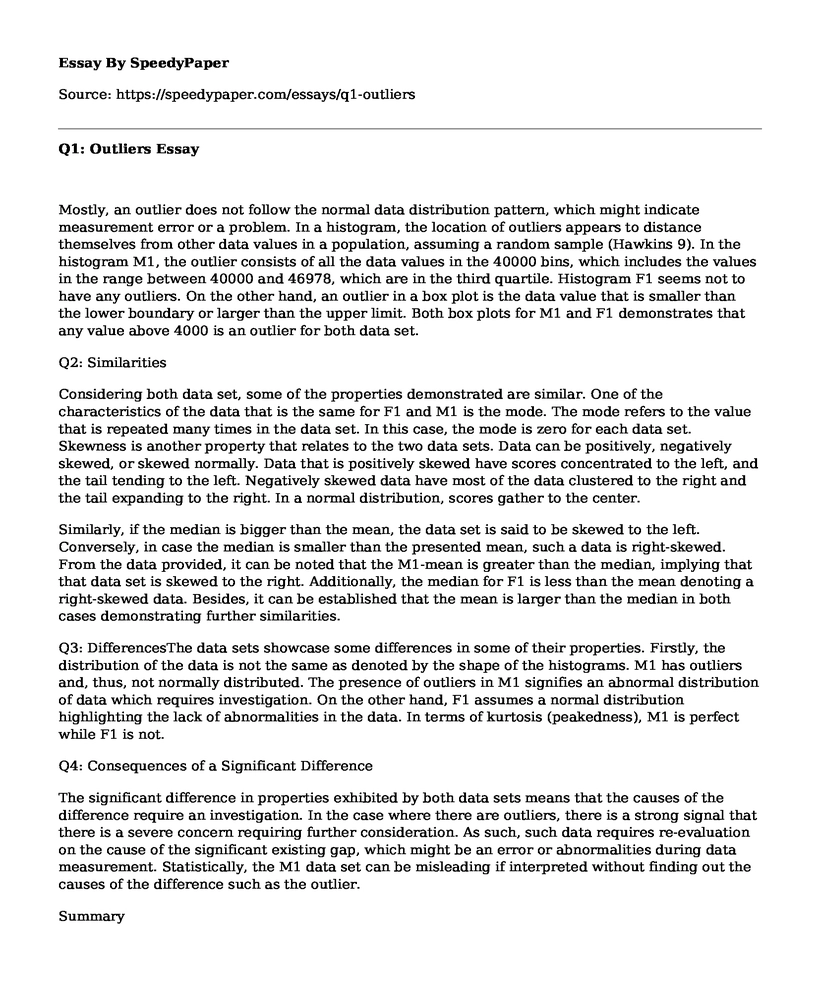# Q1: Outliers

Published: 2023-02-09Type of paper: Report Categories: History Business Disorder Civil rights Writers Pages: 3 Wordcount: 628 words
143 views

Mostly, an outlier does not follow the normal data distribution pattern, which might indicate measurement error or a problem. In a histogram, the location of outliers appears to distance themselves from other data values in a population, assuming a random sample (Hawkins 9). In the histogram M1, the outlier consists of all the data values in the 40000 bins, which includes the values in the range between 40000 and 46978, which are in the third quartile. Histogram F1 seems not to have any outliers. On the other hand, an outlier in a box plot is the data value that is smaller than the lower boundary or larger than the upper limit. Both box plots for M1 and F1 demonstrates that any value above 4000 is an outlier for both data set.

Is your time best spent reading someone else’s essay? Get a 100% original essay FROM A CERTIFIED WRITER!

Q2: Similarities

Considering both data set, some of the properties demonstrated are similar. One of the characteristics of the data that is the same for F1 and M1 is the mode. The mode refers to the value that is repeated many times in the data set. In this case, the mode is zero for each data set. Skewness is another property that relates to the two data sets. Data can be positively, negatively skewed, or skewed normally. Data that is positively skewed have scores concentrated to the left, and the tail tending to the left. Negatively skewed data have most of the data clustered to the right and the tail expanding to the right. In a normal distribution, scores gather to the center.

Similarly, if the median is bigger than the mean, the data set is said to be skewed to the left. Conversely, in case the median is smaller than the presented mean, such a data is right-skewed. From the data provided, it can be noted that the M1-mean is greater than the median, implying that that data set is skewed to the right. Additionally, the median for F1 is less than the mean denoting a right-skewed data. Besides, it can be established that the mean is larger than the median in both cases demonstrating further similarities.

Q3: DifferencesThe data sets showcase some differences in some of their properties. Firstly, the distribution of the data is not the same as denoted by the shape of the histograms. M1 has outliers and, thus, not normally distributed. The presence of outliers in M1 signifies an abnormal distribution of data which requires investigation. On the other hand, F1 assumes a normal distribution highlighting the lack of abnormalities in the data. In terms of kurtosis (peakedness), M1 is perfect while F1 is not.

Q4: Consequences of a Significant Difference

The significant difference in properties exhibited by both data sets means that the causes of the difference require an investigation. In the case where there are outliers, there is a strong signal that there is a severe concern requiring further consideration. As such, such data requires re-evaluation on the cause of the significant existing gap, which might be an error or abnormalities during data measurement. Statistically, the M1 data set can be misleading if interpreted without finding out the causes of the difference such as the outlier.

Summary

Therefore, the analysis shows that the data set M1 has outliers as denoted by the diagram starting from 4000 bin meaning an error when recording data or presence of an abnormality in the data. The mode and skewness are the two similar properties in both data sets. The mode for each data set is zero, implying none of the data value appears more than once. The two data sets demonstrate negative skewness. The peakedness is dissimilar, whereby M1 shows a perfect kurtosis and F1 does not perfect.

References

Hawkins, D M. Identification of Outliers. Dordrecht: Springer Netherlands, 1980.

Appendix

Statistical Data and Presentations